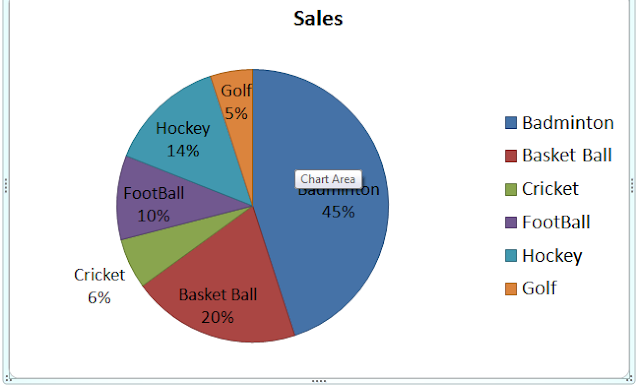New Students Offer - Use Code HELLO

# Data Interpretation Shortcuts : Techniques to Solve QuicklyTypes of Data Interpretation Questions : There are different types of Data Interpretation sets which are asked in Banking Exams like :-
• Line Graphs
• Bar Graphs
• Pie-Charts
• Table

### Why Data Interpretation Section in Banking Exams?

1. With D.I section, one can test the aspirant ability to solve statistical data.
2. In Banking Industry, there is demand of those who are highly proficient in calculation. This is because bank employees need to work on statistical data on daily basis.
3. D.I section also tests one’s ability that how quickly one can analyze the graph and extract the relevant data from it.

Topics which cover under D.I Section
There are so many topics which can cover under D.I set, like Percentage problems, Profit & Loss, Average, Age problems, Work & Time and many more…..

### Tips to Solve D.I Sets

1. You need to follow some steps while solving D.I sets in your exams in order to increase accuracy in less time. These are as follows:
2. At the very first, you need to know all the basic concepts of mathematics and also learn basic formulas as well like percentage, average and so on.
3. In Data Interpretation set, one graph is given and you need to solve some questions from the given graph. There are some variables also given and they represent some value which you need to identify.
4. You need to see the graph carefully and should try to focus on key variables.
5. In every D.I set, there are one or two questions are very simple whose answer could be obtained just by seeing the graph. So, you need to solve those questions first without wasting time on complicated questions.
6. Now, after solving easy questions you can try other which could be solve by simple formulas.
7. Please skip those questions which take heavy calculation and more time.

Let’s take a common example which could ask in exams:

### Directions:

(Q No 1-5) Study the following pie-chart carefully to answer these questions.
Total Players = 6500Q1. What is the respective ratio between number of players in hockey and number of players in Basket Ball?
a)7:9
b)7:10
c)8:11
d)7:11
e)None of These
Solution:
Total number of players = 6500 (Given)
No of players in Hockey = (14/100)*6500 = 910
No of players in Basketball = (20/100)*6500 = 1300
910/1300 = 7/10 => 7: 10

Q2. Number of players in Cricket is approximately what percentage of number of players in Football.
a)80
b)60
c)110
d)85
e)None of These
Solution: Total number of players = 6500
Number of players in Cricket = (6/100)*6500 = 390
Number of players in Football = (10/100)*6500 = 650
390 = (x/100)*650
Here we need to find value of x
X = (390/650)*100 = 60

Q3. What is the difference between number of players in Cricket and number of players in Hockey?
a)330
b)520
c)370
d)740
e)380
Solution: Total number of players = 6500(given)
Number of players in cricket = (6/100)*6500 = 390
Number of players in hockey = (14/100)*6500 = 910
Difference = 910 – 390 = 520
Q4. What is the average number of players in Football , Cricket and Basketball ?
a)880
b)990
c)780
d)800
e)900
Solution: Total number of players = 6500(Given)
Number of players in Football = (10/100)*6500 = 650
Number of players in Cricket = (6/100)*6500 = 390
Number of players in Basketball = (20/100)*6500 = 1300
Average number of players = (650+390+1300)/3 = 780

Q2. A
Q3. B
Q4. C

Try the above question after reading the article and surely it will help you to choose which question you have to do first.

Note: In short, all that you required to solve D.I set is to read the graph carefully and understand the data. Graphs could be given in many forms, either it is in Line or in Pie-Chart or in any other form also.